# Diode and Transistor NAND Gate or DTL NAND Gate and NAND Gate ICs

## Realizing NAND Gate using Diode and Transistor

For simplicity we will show here only two inputs NAND gate circuit by using diodes and transistors. This NAND gate is called DTL NAND gate or Diode Transistor Logical NAND Gate.
When both input A and B are given with 0 V, both of the diodes are in forward biased condition that is in ON condition. Supply voltage will get path to the ground through diode D1 and D2. Entire supply voltage +5 V will ideally drop across resistor R and hence base terminal of transistor T will not get enough potential to turn ON the transistor and hence the transistor will be in OFF condition. As a result supply voltage +5 V will appear at output terminal X and hence output X will become high or logical 1.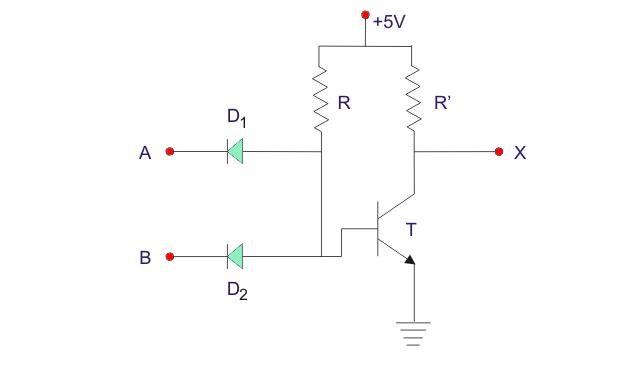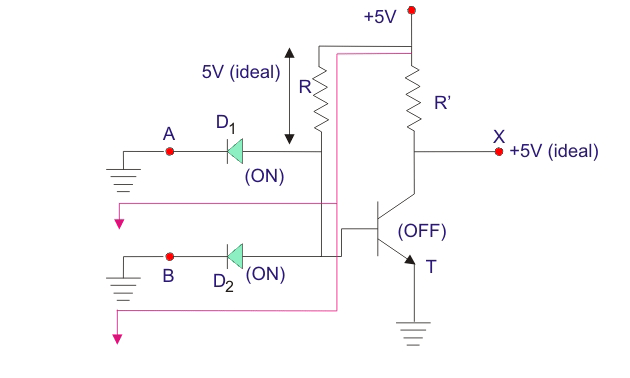Now if either of diode D1 and D2, is applied with 0 V, the same thing happens as in these cases also the supply voltage gets a path to the ground either of the forward biased diode. In that cases also the output will be logical high or 1.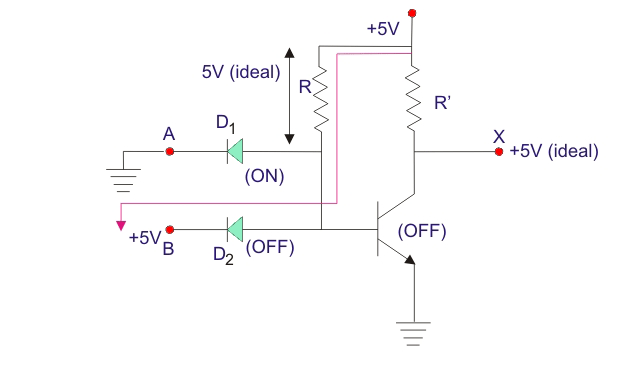When both of the inputs are given with +5 V that is logical 1, both of the diodes are in OFF condition and hence supply voltage will appear at the base terminal of the transistor T which makes it switched ON and supply voltage gets a path to the ground through this transistors. Ideally entire supply voltage +5 V will drop across resistor R’ and output terminal X will get ideally zero volts and hence the output is considered as logical 0. Hence, the output is only 0 when and only when both inputs are +5 V or logical 1.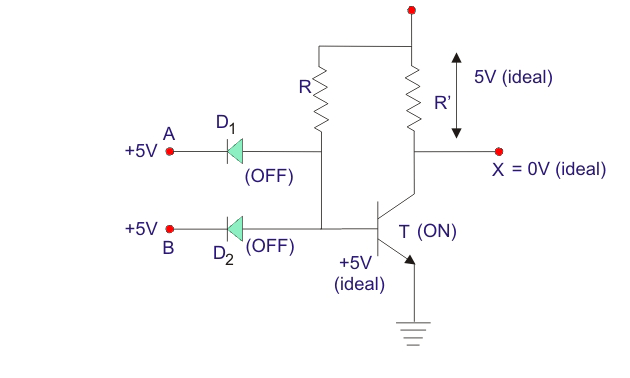## NAND Gate ICs

IC7400 is a NAND Gate IC, where four two inputs NAND gates are available. Another NAND gate IC is IC7420 which contains, two four inputs NAND gates. IC7430 contains one eight inputs NAND gates.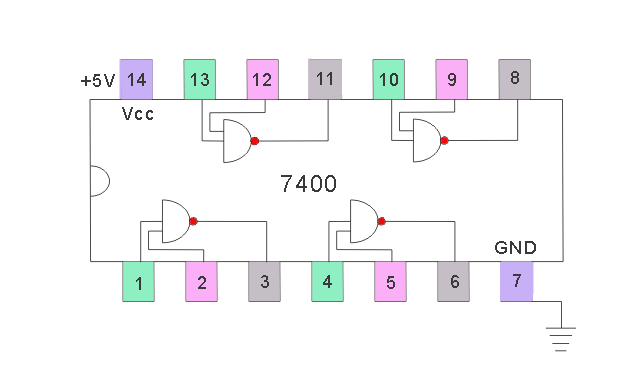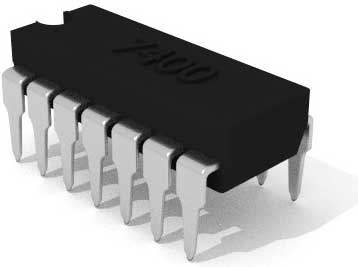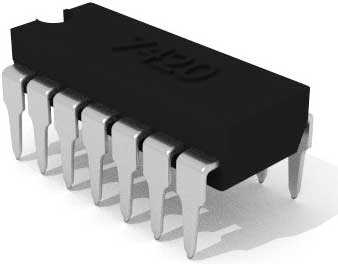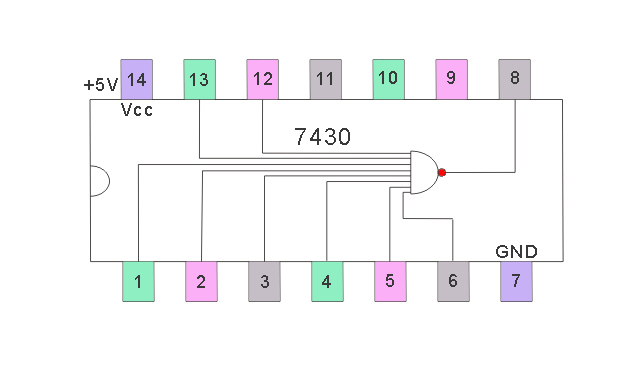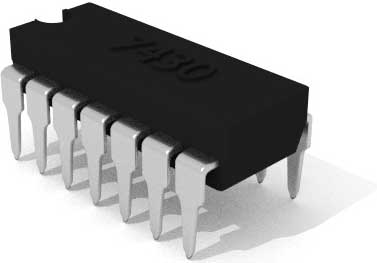Want To Learn Faster? 🎓
Get electrical articles delivered to your inbox every week.
No credit card required—it’s 100% free.